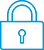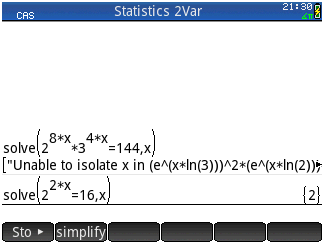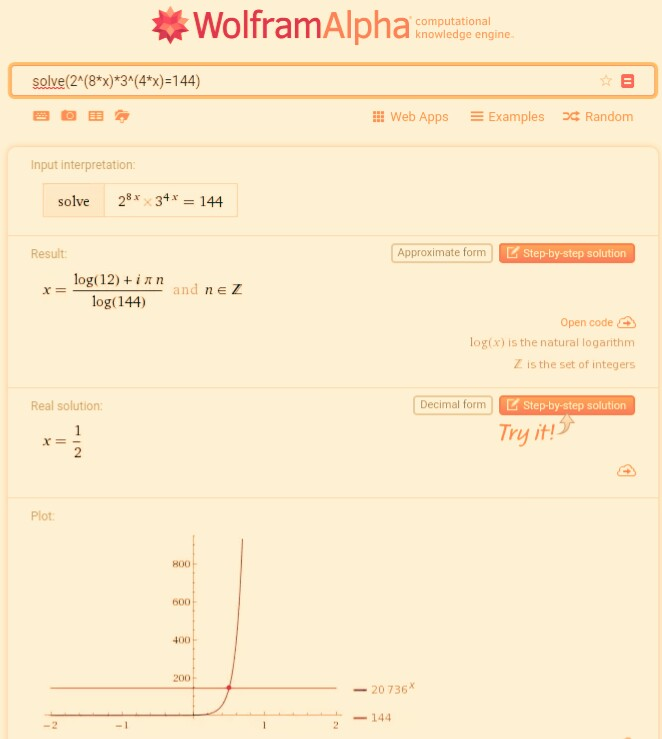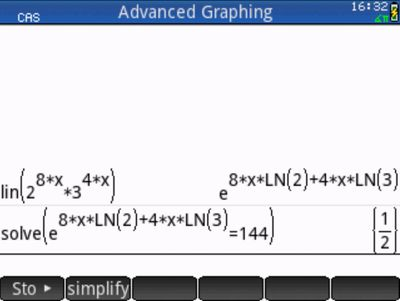cancel
Showing results for
Search instead for
Did you mean:It has been a while since anyone has replied. Simply ask a new question if you would like to start the discussion again.Level 1
8 4 0 0
Message 1 of 5
1,009
Flag Post

Solved!

# Can't solve exponential equationHP Recommended
HP PRIME

Hello!
I can't figure out, what am I doing wrong? Why calculator doesn't find that x = 1/2 is the solution?
I tried to simplify the given answer, but it didn't help.
However, it solves another much simpler equation correctly, as you can see on the picture:Help me 😞

Tags (1)
4 REPLIES 4Level 9
3,551 3,490 180 541
Message 2 of 5
Flag PostHP Recommended

Hi!, @Dopamine1:

Can you write, the equation ?.

I'm only see in the image, one light yellow triangle with gray border's.

Kind Regards !.
Have a nice day !.
@Maké (Technical Advisor Premium - HP Program Top Contributor).
Provost in HP Spanish Public Forum ... https://h30467.www3.hp.com/Level 1
8 4 0 0
Message 3 of 5
Flag PostHP Recommended

This is very strange. Here's how my post looks to me: https://pp.userapi.com/c638928/v638928620/31f54/JfM4Pxrc2Jg.jpgLevel 9
3,551 3,490 180 541
Message 4 of 5
Flag PostHP Recommended

Hi!, @Dopamine1:

See, the images ...Press, Num ... and Solve ...In comparison, with Wolfram Alpha ... http://www.wolframalpha.com/input/?i=solve(2%5E(8*x)*3%5E(4*x)%3D144)Kind Regards !.
Have a nice day !.
@Maké (Technical Advisor Premium - HP Program Top Contributor).
Provost in HP Spanish Public Forum ... https://h30467.www3.hp.com/Level 6
305 297 32 76
Message 5 of 5
Flag PostHP Recommended

@Dopamine1 wrote:

Hello!
I can't figure out, what am I doing wrong? Why calculator doesn't find that x = 1/2 is the solution?
I tried to simplify the given answer, but it didn't help.
However, it solves another much simpler equation correctly, as you can see on the picture:

The Prime can solve your equation both exactly and numerically, so approximately.

Maké showed already that you can use the Solve app for solving numerically.

Another possibility is to use fsolve instead of solve, which will solve it numerically.

Also the Advanced Graphing app is very good in solving equations numerically.

We have 2 possibilities. We can plot the graph: 2^(8*X)*3^(4*X)=144, which is the equation for a simple straight line, or we can plot 2^(8*X)*3^(4*X)-144=Y and find its zeros.

We can get the zeros in Plot view by pressing Menu – Trace – PoI – X-Intercepts.

To get them more accuretely repeatedly press the + key.

The Prime can also solve the equation exactly but needs some help.

We have to rewrite 2^(8*x)*3^(4*x) by a linearisation and then solve the equation:Tags (1)
† The opinions expressed above are the personal opinions of the authors, not of HP. By using this site, you accept the Terms of Use and Rules of Participation